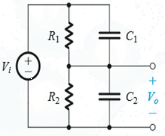Courses

# Test: Frequency Response Of Amplifier

## 10 Questions MCQ Test Electronic Devices | Test: Frequency Response Of Amplifier

Description
This mock test of Test: Frequency Response Of Amplifier for Electrical Engineering (EE) helps you for every Electrical Engineering (EE) entrance exam. This contains 10 Multiple Choice Questions for Electrical Engineering (EE) Test: Frequency Response Of Amplifier (mcq) to study with solutions a complete question bank. The solved questions answers in this Test: Frequency Response Of Amplifier quiz give you a good mix of easy questions and tough questions. Electrical Engineering (EE) students definitely take this Test: Frequency Response Of Amplifier exercise for a better result in the exam. You can find other Test: Frequency Response Of Amplifier extra questions, long questions & short questions for Electrical Engineering (EE) on EduRev as well by searching above.
QUESTION: 1

### Consider a voltage amplifier having a frequency response of the low-pass STC type with a dc gain of 60 dB and a 3-dB frequency of 1000 Hz. Then the gain db at

Solution:

Use standard formulas for frequency response and voltage gain.

QUESTION: 2

### STC networks can be classified into two categories: low-pass (LP) and high-pass (HP). Then which of the following is true?

Solution:

By definition a LP network allows dc current (or low frequency current) and an LP network does the opposite, that is, allows high frequency ac current.

QUESTION: 3

### Single-time-constant (STC) networks are those networks that are composed of, or can be reduced to

Solution:

STC has only one reactive component and one resistive component.

QUESTION: 4

The signal whose waveform is not effected by a linear circuit is

Solution:

Only sine/cosine wave are not affected by a linear circuit while all other waveforms are affected by a linear circuit.

QUESTION: 5

Which of the following is not a classification of amplifiers on the basis of their frequency response?

Solution:

None of the options provided are correct.

QUESTION: 6

General representation of the frequency response curve is called

Solution:

General representation of frequency response curves are called Bode plot. Bode plots are also called semi logarithmic plots since they have logarithmic values values on one of the axes.

QUESTION: 7

Under what condition can the circuit shown be called a compensated attenuator.​Solution:
QUESTION: 8

When a circuit is called compensated attenuator?

Solution:

Transfer function does not has frequency in its mathematical formula.

QUESTION: 9

Which of the following is true?

Solution:

Both the statements are false.

QUESTION: 10

Which of the following is true?

Solution:

These all are practical applications of different types of amplifiers.